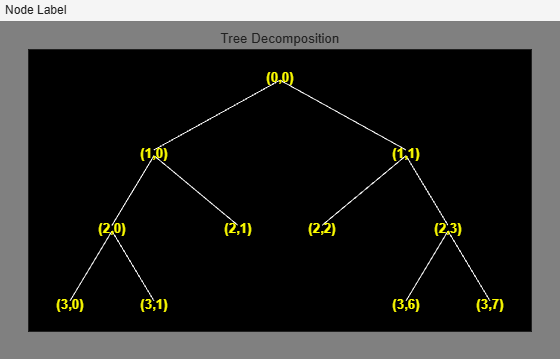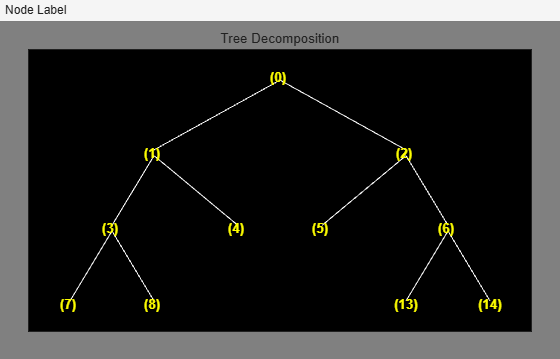# tnodes

(Not recommended) Determine terminal nodes

`tnodes` is not recommended. Use `leaves` instead.

## Syntax

``N = tnodes(T)``
``N = tnodes(T,"deppos")``
``[N,K] = tnodes(___)``

## Description

````N = tnodes(T)` returns the indices of the terminal nodes of the tree `T`. The nodes are numbered from left to right and top to bottom. The root index is 0.```

example

````N = tnodes(T,"deppos")` returns the depths and positions of the terminal nodes of the tree `T`.```
````[N,K] = tnodes(___)` returns indices `K` such that ```M = N(K)``` are the indices reordered as in the tree `T`, from left to right.```

## Examples

collapse all

Create a binary tree of depth 3.

```ord = 2; t = ntree(ord,3);```

Merge the nodes at indices 4 and 5. Plot the result.

```t = nodejoin(t,5); t = nodejoin(t,4); fig = plot(t);```Programmatically, in Node Label, change the selected item from `Depth_Position` to `Index`.

`plot(t,"setNodeLabel",fig,"Index")`List the terminal nodes as indices.

`tnodes(t)`
```ans = 6×1 4 5 7 8 13 14 ```

List the terminal nodes as depth-position.

`tnodes(t,"deppos")`
```ans = 6×2 2 1 2 2 3 0 3 1 3 6 3 7 ```

## Input Arguments

collapse all

Tree, specified as a `ntree`, `dtree`, or `wptree` object.

## Output Arguments

collapse all

Terminal node description, returned as a column vector or matrix. The nodes are numbered from left to right and top to bottom. The root index is 0.

• If `N = tnodes(T)`, `N` is a P-by-1 column vector, where P is the number of terminal nodes in the tree. `N(i)` is the index of the ith node.

• If ```N = tnodes(T,"deppos")```, `N` is a P-by-2 matrix. `N(i,1)` is the depth and `N(i,2)` is the position of the ith node.

Indices, returned as a vector. The indices `K` are such that `M = N(K)` are the terminal indices reordered from left to right.

## Version History

Introduced before R2006a